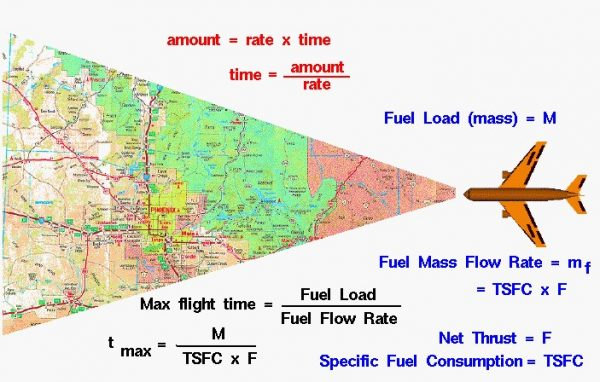# Maximum Flight Time## Aircraft Range

An airplane can cruise at a constant speed and level flight in which the lift is equal to the weight, and the thrust is equal to the drag. Since there is no net external force on the aircraft, the aircraft maintains a constant airspeed, as described by Newton’s first law of motion. The distance that the aircraft flies is then given by a simple rate equation:

### d = V * t

where d is the distance, V is the velocity and t is the time aloft. The maximum distance that the airplane can fly is called the range R:

## Maximum Time Aloft

Airplanes, unfortunately, cannot stay in the air forever. There is a time limit, or maximum time t max, that an airplane can stay aloft. The time limit is usually determined by the amount of fuel that the aircraft can carry. When the airplane runs out of fuel, the engine stops. Drag then slows the airplane, decreasing the lift. Eventually the airplane comes back to earth. We can determine maximum time available using the rate equation. The general rate equation is “rate times time equals amount”. If we do a little algebra, we can rearrange the equation to solve for the time:

### time = amount / rate

The max time for an aircraft equals the amount of fuel that we have divided by the rate that the fuel is being burned. The amount of fuel is called the fuel load and is denoted by M (given in units of kilograms or pounds mass). The rate at which the fuel is being burned is the fuel mass flow rate (given in kilograms per hour or pounds mass per hour and denoted by mf). The maximum flight time (t max) is then equal to the fuel load divided by the fuel mass flow rate

### t max = M / mf

The fuel mass flow rate depends on the type of engine used and the throttle setting chosen by the pilot. The fuel mass flow rate is related to the thrust F by a factor called the specific fuel consumption (TSFC). The specific fuel consumption equals the fuel mass flow rate divided by the thrust.

### TSFC = mf / F

Using algebra, we can determine the fuel mass flow rate:

### mf = TSFC * F

Collecting all this information, we arrive at a final equation for the maximum flight time: The maximum time aloft is equal to the fuel load divided by the specific fuel consumption times the thrust

### t max = M / (TSFC * F)

A summary of information needed to determine the range is given on a separate page.

## Aircraft Design

What does this tell us? Obviously, if we carry more fuel we can fly longer in time and farther in distance than if we carried less fuel. If our engine has a low specific fuel consumption we can also fly longer. Turboprop and turbofan engines have low specific fuel consumption and are used on long range airliners. If we can run the engine at a low throttle setting, producing a minimum amount of thrust we can also fly longer. But we must produce enough thrust to equal drag in a cruise condition. Aircraft with a low drag, or a high L/D ratio, require less thrust and can fly longer and farther than aircraft with a low L/D ratio. Aerodynamicists try to design aircraft with high L/D ratios and engines with low specific fuel consumption.

NOTE: On this page, we have taken a very simple view of aircraft range – for academic purposes. In reality, calculating the range is a complex problem because of the number of variables. An aircraft’s flight is not conducted at a single ground speed but varies from zero at takeoff, to cruise conditions, and back to zero at landing. Extra fuel is expended in climbing to altitude and in maneuvering the aircraft. The weight constantly changes as fuel is burned; so the lift, drag, and thrust (fuel consumption rate) also continually change. On real aircraft, just like with your automobile, there is usually a fuel reserve. The pilot makes sure to land the plane with fuel still on board.

Visit the Range Games interactive javascript simulation that demonstrates these concepts. The simulation presents problems that you must solve by using the range equation.

Provide feedback